Loading World Series Futures...DraftKings World Series Odds
+360
3.6 to 1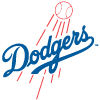Dodgers
17.0% implied probability

+700
7 to 1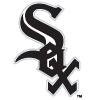White Sox
9.8% implied probability

+850
8.5 to 1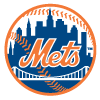Mets
8.2% implied probability

+850
8.5 to 1Padres
8.2% implied probability

+900
9 to 1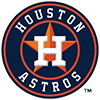Astros
7.8% implied probability

+1100
11 to 1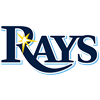Rays
6.5% implied probability

+1100
11 to 1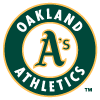Athletics
6.5% implied probability

+1400
14 to 1Yankees
5.2% implied probability

+2000
20 to 1Red Sox
3.7% implied probability

+2200
22 to 1Giants
3.4% implied probability

+2200
22 to 1Brewers
3.4% implied probability

+3300
33 to 1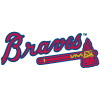Braves
2.3% implied probability

+3700
37 to 1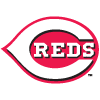Reds
2.1% implied probability

+3800
38 to 1Blue Jays
2.0% implied probability

+4000
40 to 1Indians
1.9% implied probability

+4000
40 to 1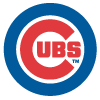Cubs
1.9% implied probability

+4000
40 to 1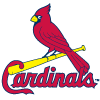Cardinals
1.9% implied probability

+4500
45 to 1Phillies
1.7% implied probability

+5000
50 to 1Angels
1.5% implied probability

+6000
60 to 1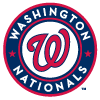Nationals
1.3% implied probability

+10000
100 to 1Twins
0.8% implied probability

+10000
100 to 1Marlins
0.8% implied probability

+10000
100 to 1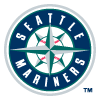Mariners
0.8% implied probability

+10000
100 to 1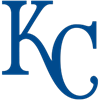Royals
0.8% implied probability

+50000
500 to 1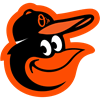Orioles
0.2% implied probability

+50000
500 to 1Diamondbacks
0.2% implied probability

+100000
1000 to 1Pirates
0.1% implied probability

+100000
1000 to 1Tigers
0.1% implied probability

+100000
1000 to 1Rockies
0.1% implied probability

+100000
1000 to 1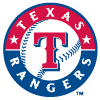Rangers
0.1% implied probability